# Radiation & Medical Physics

Mary PW Chin 钱碧慧博士
PhD (Wales), MSc (Surrey)
E-mail:

#### Intro to image processing

Digital photos -> medical images☞ slides

#### Intro to radiotherapy

Geography -> dosimetry☞ slides

#### Photon interactions I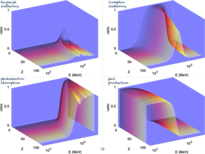☞ slides

#### Photon interactions II☞ slides

#### Photon interactions III☞ slides

#### How hadrontherapy works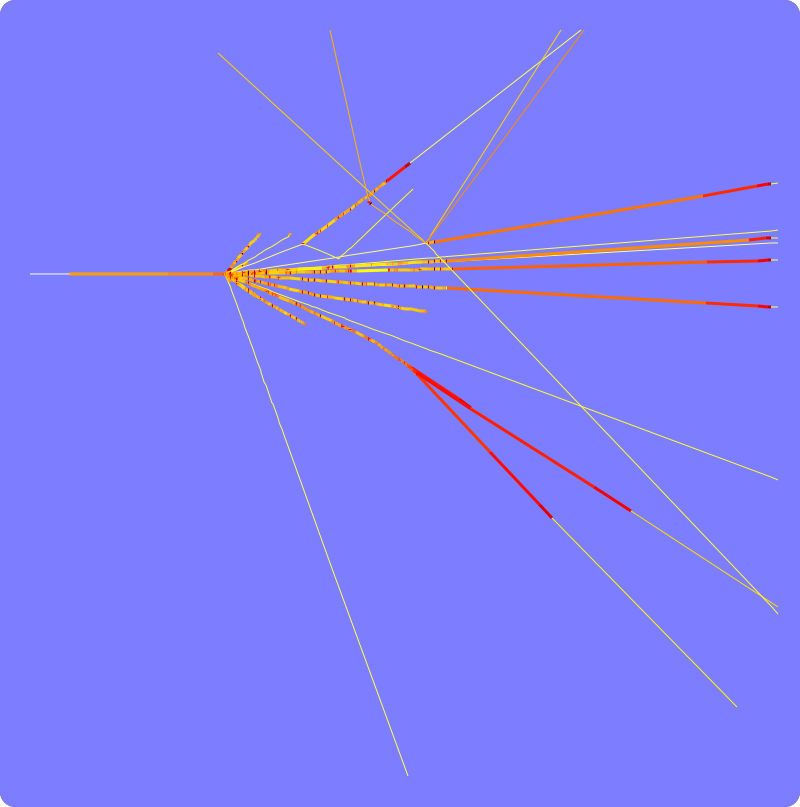#### How spallation works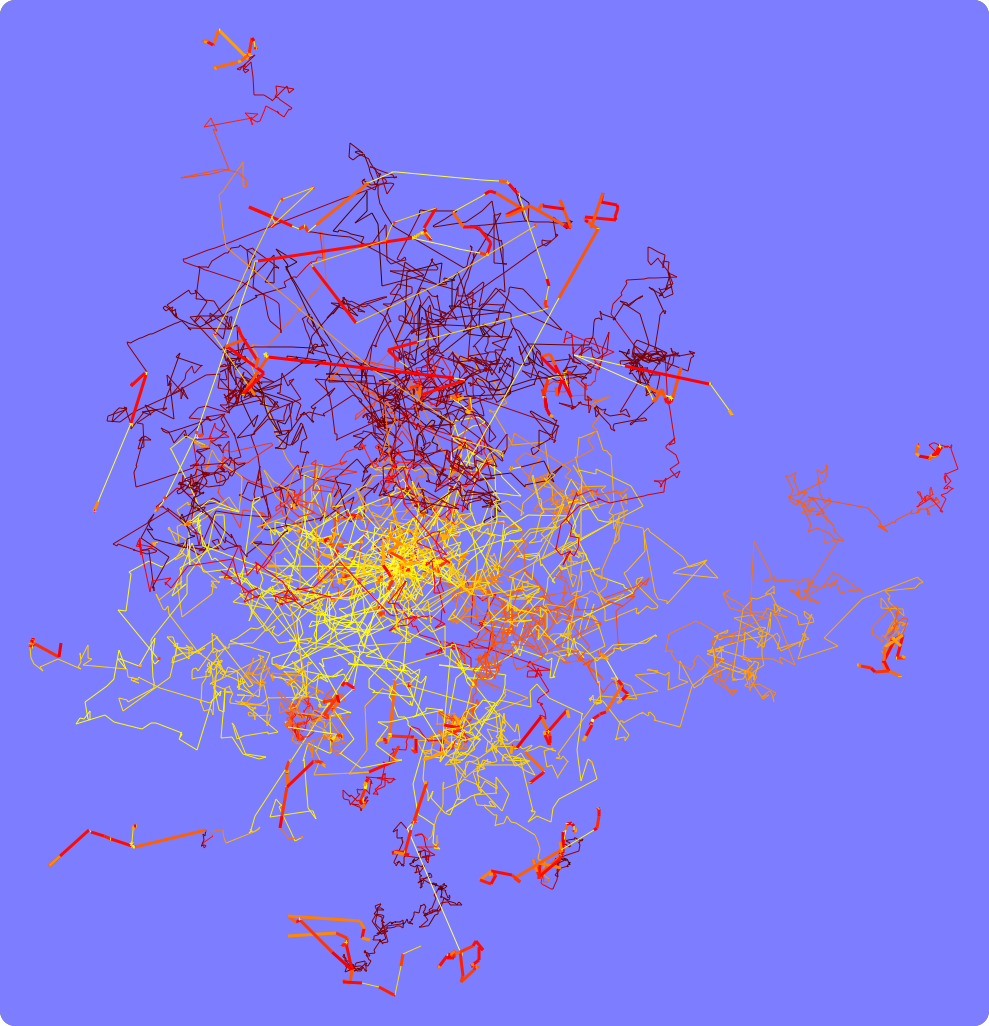#### Laboratory #1

Derive CT numbers

#### Laboratory #2

Map photon interaction dominance

#### Questions for revision

Medical application of ionising radiation

#### Monte Carlo from scratch☞ slideshow

#### FLUKA tutorial☞ slideshow

Laboratory #1: derive CT numbers
##### Part A: Body tissues
1. To derive HU, first we need find μwater and μ for each material we wish to derive HU for.
2. We are going to lookup the XCOM database for μwater and μ.
3. Before giving us the answer, XCOM will first ask us for
• the elemental composition of the material we want the μ for.
• the energy of the photons from the CT machine. Refer to the CT chapter by your favourite author, convince yourself that 80 to 120 keV is about the reasonable approximate.
4. From the NIST tissue composition database: extract both data columns: the atomic number and the fractional weight. Translate the atomic number in the first column to element names, e.g. H, C, N, … In case you need help, refer to the periodic table.
ICRP lung.
fractional weight
H 0.101278
C 0.102310
N 0.028650
O 0.757072
Na 0.001840
Mg 0.000730
P 0.000800
S 0.002250
Cl 0.002660
K 0.001940
Ca 0.000090
Fe 0.000370
Zn 0.000010
ICRP cortical bone.
fractional weight
H 0.047234
C 0.144330
N 0.041990
O 0.446096
Mg 0.002200
P 0.104970
S 0.003150
Ca 0.209930
Zn 0.000100
5. Go to NIST's XCOM online database; select 'Mixture' and click 'Submit Information'.
6. Paste the data (in pure text format) you obtained from step 4 into the window asking for relative weights. This window does not like tabs, so please remove any tabs.
7. Click 'Submit Information'. In return, expect to get a graph followed by a table.
8. Locate the row in the table where the 2nd column (Photon Energy) says 8.000E-02 MeV (80 keV). Along the same row, take the value in the column under 'Total Attenuation with Coherent Scattering'. This is the mass attenuation coefficient (μ/ρ) at 80 keV for the material at hand.
9. You should get 1.824E-01 cm2/g and 2.221E-01 cm2/g for lung and cortical bone, respectively.
10. We still need to find μwater. For this, go back to NIST's XCOM online database; select 'Compound' and click 'Submit Information'.
11. Enter 'H2O' in the window asking for 'Formula for compound'. Click 'Submit Information'. Repeat step 8. You should get 1.837E-01 cm2/g for water.
12. The mass attenuation coefficient (μ/ρ) is independent of density and is therefore a more fundamental property than the linear attenuation coefficient (μ). Do not be misled by the presence of 'gram' in its unit (cm2/g), mistaking it as carrying density effects embedded within. The linear attenuation coefficient (μ) is the one which depends on density; to make it independent of density we divide μ by the density and this is how that 'gram' in μ/ρ came to be. We know that CT scanning, and therefore HU, is a measure of density (as well as the elemental composition). HU therefore cannot be independent of density. So, Equation 1 rightly asks for μ, not μ/ρ.
13. We are now ready to convert μ/ρ to μ by multiplying with the density. Let us use 0.260 g/cm3 and 1.850 g/cm3 as density for lung and cortical bone, respectively.
1.837E-01 cm2/g × 1.000 g/cm3 = 1.837E-01 cm-1 [water]
1.824E-01 cm2/g × 0.260 g/cm3 = 0.474E-02 cm-1 [lung]
2.221E-01 cm2/g × 1.850 g/cm3 = 4.109E-01 cm-1 [cortical bone]
14. We are finally ready for Equation 1:
HU = 1000 ( 1.915E-01 - 1.837E-01 ) / 1.837E-01 = -742 [lung]
HU = 1000 ( 4.109E-01 - 1.837E-01 ) / 1.837E-01 = 1237 [cortical bone]
##### Part B: Contrast agents
15. Now let us derive the HU for barium and iodine.
16. Go back to NIST's XCOM online database; select 'Element' and click 'Submit Information'.
17. Enter 'Ba' in the window asking for 'Symbol'; click 'Submit Information'. Repeat step 8 to grab μ/ρ for barium at 8.000E-02 MeV.
18. Go back to the previous page where the form asks for the 'Symbol' for an element. Enter 'I' in the in the window asking for 'Symbol'; click 'Submit Information'. Repeat step 8 to grab μ/ρ for iodine at 8.000E-02 MeV.
19. We should get μ/ρ = 3.963 cm2/g and 3.510 cm2/g, respectively.
20. Applying ρ = 3.500 g/cm3 and 4.930 g/cm3 for barium and iodine, respectively, we get:
HU = 1000 ( 3.963 × 3.500 - 1.837E-01 ) / 1.837E-01 = 74506 [barium]
HU = 1000 ( 3.510 × 4.930 - 1.837E-01 ) / 1.837E-01 = 93199 [iodine]

This explains how barium and iodine act as CT contrast agents, causing the pixels to be very bright, differentiating enhanced regions from other tissues. Note that barium has a higher μ/ρ than iodine, but a lower ρ, effectively a lower μ. Iodine ends up a stronger contrast agent.
##### Part C: Megavoltage imaging
21. Repeat steps 1 to 14 for 2 MeV instead of 80 keV. We find that HU at 2 MeV for cortical bone is now 746 rather than 1237. The difference between cortical bone and water is therefore 1237 at 80 keV and 746 at 2 MeV. This reduction in contrast is a numeric demonstration of the undesirable feature of megavoltage CT, which operates at photon energies optimised for therapy rather than imaging.
A summary of our exercise, with some bonus materials included.
ρ (g/cm3) μ/ρ (cm2/g) HU
80 keV2 MeV80 keV2 MeV
water 1.00 1.837E-01 4.942E-02 0 0
lung 0.26 1.824E-01 4.893E-02 -742-743
cortical bone 1.85 2.221E-01 4.663E-02 1237 746
barium 3.50 3.963E+00 4.078E-02 74506 1888
iodine 4.93 3.510E+00 4.124E-02 93199 3114
air 1.20479E-03 1.650E-01 4.452E-02 -1000 -1000
adipose 0.92 1.806E-01 4.963E-02 -96 -76
blood 1.06 1.824E-01 4.896E-02 52 50
brain 1.03 1.838E-01 4.933E-02 31 28
compact bone 1.85 2.087E-01 4.733E-02 1102 772
##### Endnote

The calculations above are estimations. CT numbers are never exactly so. Uncertainties include variations in density and most importantly, tissue composition. We have used the tissue composition provided by ICRP. Measuring elemental composition is a highly specialised field of its own, with many challenges. Samples have inevitably been in-vitro, if not cadavers'.

Laboratory #2: map photon interaction dominance

Does this plot look familiar? It appears in every textbook on radiation physics. What is x axis? What is y axis? What are their units?

X-axis is in energy (MeV). Y-axis is the atomic number (Z). The plot shows the dominant interaction type for different elements at different energies. The three competing interaction types are photoelectric absorption, Compton scatter and pair production.

1. Go to NIST's XCOM database for elements.
2. In the window asking for 'Atomic Number' enter '1'. Click 'Submit Information'.
3. We should get a new page showing a graph followed by a table.
4. In the table, check the tick boxes on the header row for 'Coherent', 'Incoherent', 'Photoeletric Absorption', 'In Nuclear Field', 'In Electron Field' and 'With Coherent Scattering'.
6. Once a new page containing columns of data appears, use your browser to save the file (usually Ctrl-S works).
7. Repeat steps 1 to 6 for atomic numbers up to, say, 70. It is your choice whether to download every step of Z (Z = 1, 2, 3, …, your graph will look nicer) or to download at intervals of Z (Z = 1, 10, 20, 30, …, your graph shall have data points spaced apart).
8. We now have a collection of text files saved, each containing the photon μ/ρ for different elements. Each file begins with 3 header rows. Data start from the 4th row. The 1st column is the energy (MeV). The remaining columns contain the μ/ρ for different interaction types, including the three main types of interest to us: Compton scattering ('Incoherent'), photoelectric absorption and pair production ('In Nuclear Field' plus 'In Electron Field').
9. Each file (corresponding to the respective Z) we downloaded will contribute two data points towards constructing our own version of Figure 1.
• E1: the energy where photoelectric absorption passes over its dominance to Compton scattering;
• E2: the energy where Compton scattering passes over its dominance to pair production.
That is to say:
• at E < E1, photoelectric cross section exceeds those of Compton scattering and pair production;
• within the range E1 < E < E2, Compton scattering cross section exceeds those of photoelectric absorption and pair production;
• at E > E2, pair production cross section exceeds those of photoelectric absorption a nd Compton scattering.
10. Finding the two data points row by row for each file would not only be tedious but error prone. Manual handling is not the way. So, let's automate the process. In what follows, I provide a multi-lingual solution, whichever programming language you prefer: Except Matlab, all are freely downloadable. Octave is ala-Matlab, but free; most functions work equally well. Python, Perl and GNUplot usually come packaged with the Linux operating system already. Programming languages differ by syntax, but the algorithm to complete a given task is the same no matter which language is it implemented with.
11. Putting on our computing hat, here is the input we need to process/crunch/compute in order to produce the output we desire (that is, Figure 2):
• Input: a collection of files (downloaded from step 6), one for each element. The files are in pure text format (as opposed to the binary format and the rich text format). Each file contains:
• two rows of text headers;
• a blank row;
• many rows of spaced-delimited numbers, where the energy increases row by row;
• 7 columns to each row: the first column is the energy in MeV, the remaining columns are the μ/ρ for different components.
• Process:
• scan each file row by row;
• compare μ/ρ for photoelectric effect (column 4), Compton (column 3) and pair production (column 5 plus column 6, which are components from the nuclear and electron fields, respectively);
• find the dominant interaction for each row (i.e. for each energy);
• find the energy where the dominance switches from photoelectric to Compton, and where the dominance switches from Compton to pair production.
• Output:
• (optional) save the data we obtained in three columns: the atomic number (Z), the energy where the dominance switches from photoelectric to Compton, and the energy where the dominance switches from Compton to pair production.
• plot the data we obtained, in the spirit of Figure 1. That is, Z on the vertical axis and energy on the horizontal axis, which should be set to the logarithmic scale. This would produce Figure 2.
12. Taking a level down, closer to the computer's psyche rather than ours, the tasks outlined in step 12 translate into the following algorithm, which is exactly what the three program listings (Matlab/Octave, Python, Perl+GNUplot) do.
````open a new file for output                              `
`scan the directory for input files                      `
`loop over each input file                               `
`  initialise user variable                              `
`  decipher Z from the filename                          `
`  write Z on the output file                            `
`  open the input file                                   `
`  loop over each row in this input file                 `
`    read the energy                                     `
`    read μ/ρ for Compton scatter                `
`    read μ/ρ for photoelectric absorption       `
`    read and compute μ/ρ for pair production    `
`    if photoelectric dominates, set thisflag to 1      `
`    if Compton scatter dominates, set thisflag to 2    `
`    if pair production dominates, set thisflag to 3    `
`    if dominance switches from photoelectric to Compton,`
`      record the energy on the output file              `
`    if dominance switches from Compton to pair,         `
`      record the energy on the output file		    `
`    in case of other switches of dominance,             `
`      announce the unexpected on the screen             `
`    remember thisflag by storing as lastflag            `
`finished writing, close the output file                 `
`read the file we have just written                      `
`set graph to superposition mode                         `
`plot (E,Z) where E is the energy where dominance switches  `
`set horizontal axis to log                              `
`print the legend                                        `
`label the axis                                          `
`turn grid on                                            `
```
Listing 1. The algorithm.
13. To track the switch of dominance, I use two variables: thisflag (for the current row) and lastflag (to remember the previous row). They take on the value 1 if photoelectric absorption is dominant, 2 if Compton scattering is dominant, 3 if pair production is dominant.
14. Note that I saved the files from step 6 as 01.txt, 02.txt, 03.txt … for hydrogen, helium, lithium, … According to your file-naming convention, you probably need to modify line 2 of Listing 2 (for Matlab/Octave), line 6 of Listing 3 (for Python), line 2 of Listing 4 (for Perl).
15. Whereas Matlab/Octave and Python (assuming Matplotlib is installed) readily plot graphs within the same environment, Perl doesn't. Perl is excellent for data handling though. Output can be easily written to file, which can be readily plotted using GNUplot. This is exactly the option I provide here.
16. For consistency between the three programming options provided here, I opt to write the output to a file anyway — even when ready plotting is available within the same environment. I also opt to read from file the data to be plotted, for the sake of consistency. I could have stored the data in memory as an array instead.
17. I have colour-coded all 4 program listings as a learning aid to readers new to programming. I encourage beginners to try colour-coding whatever program listings they encounter; this will provide a leg up on programming.
• Built-in commands intrinsic of the programming language appear in blue. These we can't change as we like, or we knock the program out of order.
• Variables defined by the user appear in red. These we can change according to our liking. Take Listing 1, for example, we can replace all occurrences of out to donkey, alice or smile, and the program will still sing and dance all the same. The worse we could do would be to confuse ourselves, wondering what is the donkey's role here or what is Alice doing here. So, best to name variables meaningfully — when you revisit the program next time, or if you pass the program to someone else, your program will be less painful to decrypt.
• Instantaneous values appear in limegreen. In computing terms, values may be numbers or characters.
````out = fopen('dominance.mout','w');`
`file = dir('*.txt');`
`for f = 1:numel(file)`
`  lastflag = 0;`
`  field = strsplit(file(f).name,'.');`
`  Z = str2num(field{1});`
`  fprintf(out,'%d ',Z);`

`  a = importdata(file(f).name,' ',3);`
`  for row = 1:size(a.data,1)`
`    E = a.data(row,1);`
`    compt = a.data(row,3);`
`    photo = a.data(row,4);`
`    pairp = a.data(row,5) + a.data(row,6);`
`    if (photo>compt && photo>pairp)`
`      thisflag = 1;`
`    elseif (compt>photo && compt>pairp)`
`      thisflag = 2;`
`    elseif (pairp>photo && pairp>compt)`
`      thisflag = 3;`
`    end`
`    if (lastflag==1 && thisflag==2)`
`      fprintf(out,'%.3e ', E);`
`    elseif (lastflag==2 && thisflag==3)`
`      fprintf(out,'%.3e\n', E);`
`    elseif (thisflag~=lastflag && lastflag>0)`
`      fprintf(out,'Exception! %.3e %d\n', E, Z);`
`    end`
`    lastflag = thisflag;`
`  end`
`end`
`fclose(out);`

`b = load('dominance.mout');`
`hold on`
`plot(b(:,2),b(:,1),'r.')`
`plot(b(:,3),b(:,1),'bx')`
`set(gca,'xscale','log')`
`legend('Photoelectric to Compton','Compton to Pair','location','northwest')`
`legend boxoff`
`xlabel('Photon energy (MeV)')`
`ylabel('Atomic number')`
`grid on`
```
Listing 2. Matlab script for this exercise; works equally well in Octave. ☞ download
````import glob`
`import numpy as np`
`import matplotlib.pyplot as plt`

`out = open("dominance.pyout","w")`
`for file in glob.glob("[0-9]*.txt"):`
`  lastflag = 0`
`  field = file.split(".")`
`  Z = int(field)`
`  out.write("%d " % Z)`
`  `
`  with open(file) as xcom:`
`    for tisline in xcom.readlines()[3:]:`
`      field = tisline.split()`
`      E = float(field)`
`      compt = float(field)`
`      photo = float(field)`
`      pairp = float(field)+float(field)`
`      if (photo>compt and photo>pairp) :`
`        thisflag = 1`
`      elif (compt>photo and compt>pairp) :`
`        thisflag = 2`
`      elif (pairp>photo and pairp>compt) :`
`        thisflag = 3`
`      if (lastflag==1 and thisflag==2) :`
`        out.write("%.3e " % E)`
`      elif (lastflag==2 and thisflag==3) :`
`        out.write("%.3e\n" % E)`
`      elif (thisflag!=lastflag and lastflag>0) :`
`        out.write("Exception! %.3e %d\n" % (E,Z))`
`      lastflag = thisflag`
`out.close()`

`b = np.loadtxt("dominance.pyout")`
`plt.plot(b[:,1],b[:,0],'r.')`
`plt.plot(b[:,2],b[:,0],'bx')`
`plt.gca().set_xscale('log')`
`plt.xlabel('photon energy (MeV)')`
`plt.ylabel('Atomic number')`
`plt.grid(True)`
`plt.show()`
```
Listing 3. Python script for this exercise. ☞ download
````open(out,">dominance.plout");`
`while(defined( \$file=glob("[0-9]*.txt") )) {`
`  open(xcom,\$file);`
`  \$lastflag = 0;`
`  @field = split /\./, \$file;`
`  \$Z = \$field;`
`  printf out "%d ",\$Z;`
`  <xcom>; <xcom>; <xcom>; `
`  `
`  while(\$thisline = <xcom>) {`
`    @field = split /\s+/, \$thisline; `
`    \$E = \$field;`
`    \$compt = \$field;`
`    \$photo = \$field;`
`    \$pairp = \$field + \$field;`
`    if (\$photo>\$compt && \$photo>\$pairp) {`
`      \$thisflag = 1;`
`    }`
`    elsif (\$compt>\$photo && \$compt>\$pairp) {`
`      \$thisflag = 2;`
`    }`
`    elsif (\$pairp>\$photo && \$pairp>\$compt){`
`      \$thisflag = 3;`
`    }`
`    if (\$lastflag==1 && \$thisflag==2) {`
`      printf out "%.3e ",\$E;`
`    }`
`    elsif (\$lastflag==2 && \$thisflag==3) {`
`      printf out "%.3e\n",\$E;`
`    }`
`    elsif (\$thisflag!=\$lastflag && \$lastflag>0) {`
`      printf "Exception! %.3e %d\n",\$E,\$Z;`
`    }`
`    \$lastflag = \$thisflag;`
`  }`
`}`
`close(out);`
```
Listing 4. Perl script for this exercise. ☞ download
````set logscale x`
`set xlabel "Photon energy (MeV)"`
`set ylabel "Atomic number"`
`set key left top`
`set grid`
`plot "dominance.plout" using 2:1 title 'Photoeletric to Compton', \`
`     "dominance.plout" using 3:1 title 'Compton to Pair'`
```
Listing 5. GNUplot script for this exercise. ☞ download
Dominance of photon cross sections for Z = 20.
energy rangedominant interaction
E < 0.1 MeV photelectric absorption
0.1 MeV < E < 12 MeV Compton scattering
E > 12 MeV pair production
18. Voila, we should then be able to plot from our own produce (Figure 2) a graph similar to Figure 1.
19. Pick a point on the graph, for instance Z = 20 and E = 0.1 MeV, where the curve separates photoelectric dominance (left of the curve) from Compton dominance (right of the curve). That means for Z = 20 (which is calcium), photons at energies up to 0.1 MeV will most likely undergo photoelectric absorption, dominating over Compton scatter and pair production. Compton scattering and pair production may still occur, but less likely compared to photoelectric absorption. If we send a 0.09 MeV photon into a sea of calcium, it may undergo photoelectric absorption, Compton scattering or pair production; I wouldn't bet on a single photon. But if we send in a thousand of these photons, I may then safely bet that the majority of the interactions would be photoelectric absorption.

Along Z=20 on the same plot, there is a second curve intersecting at E = 12 MeV. In combination with the first intersection (E = 0.1 MeV), the energy domain is divided into three sections, as shwown in the table, each dominated by one of the three competing interaction types.

Matlab/Octave, Python, Perl, GNUplot side-by-side

Whether for drilling or learning, it would be fun to put the three programming solutions (Listing 2, Listing 3, Listing 4) from the preceding section side by side to see how the corresponding syntax and commands compare.

##### Philosophy
• Matlab is most user-friendly of the lot.
• Python is most modular by philosophy, hence the preamble of imports (lines 1 to 3 in Listing 3) without which the program will not run. No preamble is necessary for Listing 2 (Matlab/Octave) and Listing 4 (Perl).
• Perl is simply and elegantly Perl; codes can be short and brief yet so powerful.
##### Syntax
• Python economises by doing without Matlab's end and Perl's }. How then does Python recognise where a block ends? From the indents; it disciplines programmers into a fixed indenting rule.
• The semicolon ; at the end of each line is optional in Matlab but not in Perl. Yes, it is optional in Matlab but removing the semicolons would cost us unending screen dumps. As a matter of habit we usually remove the semicolon only very selectively, normally during debugging, as a lazy alternative to writing additional lines to display values on the screen.
• A dollar sign leads every user variable in Perl; neither Matlab nor Python is currency minded.
• When a line is too long, typing to a continuation line (with full awareness by the programming language) takes three dots in Matlab and a blackslash in GNUplot. Python accepts a blackslash too, but it usually figure out whether a line is continued or new from its strict indentation policy anyway.
##### Commands
• fopen and fclose are the commands for opening and closing files. Python and Perl drop the leading 'f' and use open and close instead. In fact in Perl I customarily use open(…) || die "oopsy can't open file" to catch the exception (using Java's nomenclature) in case of error; this open-or-die line is very handy to aid graceful error handling.
• We are encouraged to read files without opening the file explicitly (which would require explicit closing of the file, which programmers sometimes forget). Reading without explicit opening is made possible by Python's with open(file) as, which ensures automatic, graceful closing of the file. Matlab's load command is most minimalistic but it can only handle files containing only numerics (not characters) and it requires that all lines have the same number of columns. Since our exercise in the previous section involves files containing headers, I have used importdata instead of load.
• I pass 3 as an argument to Matlab's importdata (line 9 of Listing 2) and in Python I slice the array by 3 onwards (line 13 of Listing 3), to alert the program of 3 lines of headers.
• I have used Matlab's dir command in place of Python's and Perl's glob to do a directory sweep, which saves me from naming the input files one by one.
• Splitting of a delimited string into an array is accomplished via strsplit (Matlab) and split (Python and Perl).
• else if is spelled elseif, elif and elsif in Matlab, Phython and Perl, respectively.
Matlab/Octave, Python & Perl: file and data handling.
Matlab/OctavePythonPerl
import glob
import numpy as np
import matplotlib.pyplot as plt
out=fopen('dominance.mout','w');

fclose(out);
out = open("dominance.pyout","w")

out.close()
open(out,"> dominance.plout");

close(out);
file = dir('*.txt');
for f = 1:numel(file)

end
for file in glob.glob("*.txt"): while(defined( \$file=glob("*.txt") )) {

}
field = strsplit(file(f).name,'.');
Z = str2num(field{1});
field = file.split(".")
Z = int(field)
@field = split /\./, \$file;
\$Z = \$field;
fprintf(out,'%d ',Z); out.write("%d " % Z) printf out "%d ",\$Z;
a = importdata(file(f).name,' ',3);
for row = 1:size(a.data,1)
with open(file) as xcom:
for tisline in xcom.readlines()[3:]:
open(xcom,\$file);
<xcom>; <xcom>; <xcom>;
while(\$thisline = ) {
E = a.data(row,1); E = float(field) \$E = \$field;
if (photo>compt && photo>pairp)

elseif (compt>photo && compt>pairp)

end
if (photo>compt and photo>pairp) :

elif (compt>photo and compt>pairp) :
if (\$photo>\$compt && \$photo>\$pairp) {

} elsif (\$compt>\$photo && \$compt>\$pairp) {

}
Matlab/Octave, Python & GNUplot: plotting.
Matlab/OctavePythonGNUPlot
hold on
plot(b(:,2),b(:,1),'r.')
plot(b(:,3),b(:,1),'bx')
set(gca,'xscale','log')
legend('Photoelectric to Compton',...
'Compton to Pair',...
'location','northwest')
legend boxoff
xlabel('Photon energy (MeV)')
ylabel('Atomic number')
grid on
plt.plot(b[:,1],b[:,0],'r.')
plt.plot(b[:,2],b[:,0],'bx')
plt.gca().set_xscale('log')
plt.xlabel('Photon energy (MeV)')
plt.ylabel('Atomic number')
plt.grid(True)
plt.show()
set logscale x
set xlabel "Photon energy (MeV)"
set ylabel "Atomic number"
set key left top
set grid
plot "dominance.plout" using 2:1 \
title 'Photoeletric to Compton',\
"dominance.plout" using 3:1 \
title 'Compton to Pair'
Questions for revision
1. Medical accelerators conventionally deliver 6 MV and 10 MV photon beams. In the patient's body, energy is deposited by
photons only
electrons only
photons and electrons
neither photons nor electrons
2. During commissioning and quality assurance for radiotherapy, a water phantom is so frequently used to represent the human body because
over 50% of an average adult human body is water
ion chambers are calibrated for measurements in water
radiation properties of the human body approximate those of water
density of the human body approximates that of water
3. The ideal dose distribution on a treatment plan would be
100% perfectly uniform throughout the outer target volume, zero outside
97%% perfectly uniform throughout the outer target volume, zero outside
95%% perfectly uniform throughout the outer target volume, zero outside
varying according to local biological response in the target volume, zero outside
4. Neutron contamination is present when a linac operates in the following modes
6 MV and above
10 MV and above
18 MV and above
all electron modes
5. In BNCT the therapeutic dose is delivered by
alphas and lithiums
alphas only
neutrons
electrons
6. The Bragg peak drops abruptly at the far extent of the target volume. This is principle underlying
BNCT
Tomotherapy
Cyberknife
7. In bunker design, walls and mazes play the following principal roles:
walls reduce penetration, mazes reduce scatter
walls reduce scatter, mazes reduce penetration
walls reduce both penetration and scatter, mazes do not reduce the dose outside
walls and mazes reduce penetration and scatter all the same
8. In proton therapy, peak-to-entrance dose ratios between a pencil beam and a SOBP beam
differ significantly
differ negligibly
equal exactly
differ significantly or not, depending on body site
9. The mass attenuation coefficient
is density dependent
is the inverse of linear attenuation coefficient
provides a measure of tissue mass
none of the above is correct
10. For radiation protection around a megavoltage linac, the most desirable interaction would be
Compton scattering
positron annihilation
pair production
photoelectric effect
11. Particles which are directly ionising include
electrons and protons but not photons and neutrons
electrons, protons and photons but not neutrons
electrons, protons, photons and neutrons
electrons only
12. On a treatment plan typically we expect envelops of increasing areas
GTV < CTV < PTV
PTV < CTV < GTV
CTV < PTV < GTV
PTV < GTV < CTV
13. A bolus is in contact with the skin; a compensator is attached to the treatment unit.
Skin sparing is considerable amd comparable in both cases.
Skin sparing is achieved with a bolus, given the direct contact.
Skin sparing is achieved with a compensator, given the large air gap.
Skin sparing is lost in both cases.
14. Dose computations from convolution techniques and Monte Carlo simulations differ most
in the head and neck area
in the thorax
in the abdomen
in the limbs
15. During PET examinations the patient is injected with a positron-emitting radioisotope e.g. 18F-FDG. Signal for image construction relies on coincident gamma pairs. A coincident gamma pair is a product of positron annihilation with an electron. Where does the electron come from?
atoms within the patient's body
the PET scanner
the radioisotope supplies both positrons and electrons
none of the above
16. Which of the following windows or filters are always in operation during PET?
time
energy
angle
all of the above
17. How do Figure 3 and Figure 4 differ?

## Computer programming

#### Python programming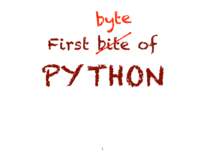☞ slides

#### Matlab / Octave☞ slides

#### Perl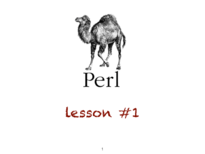☞ slides

#### C☞ slides

#### Multilingual programming

Matlab, Octave, Python, Perl, GNUplot

#### Linux

Command-line Linux☞ slides

#### HTML & CSS

Build your own website☞ slides

#### LaTeX☞ slides

#### Meeting of Nobel Laureates

☞ 55th Lindau Meeting

Worm Atlas